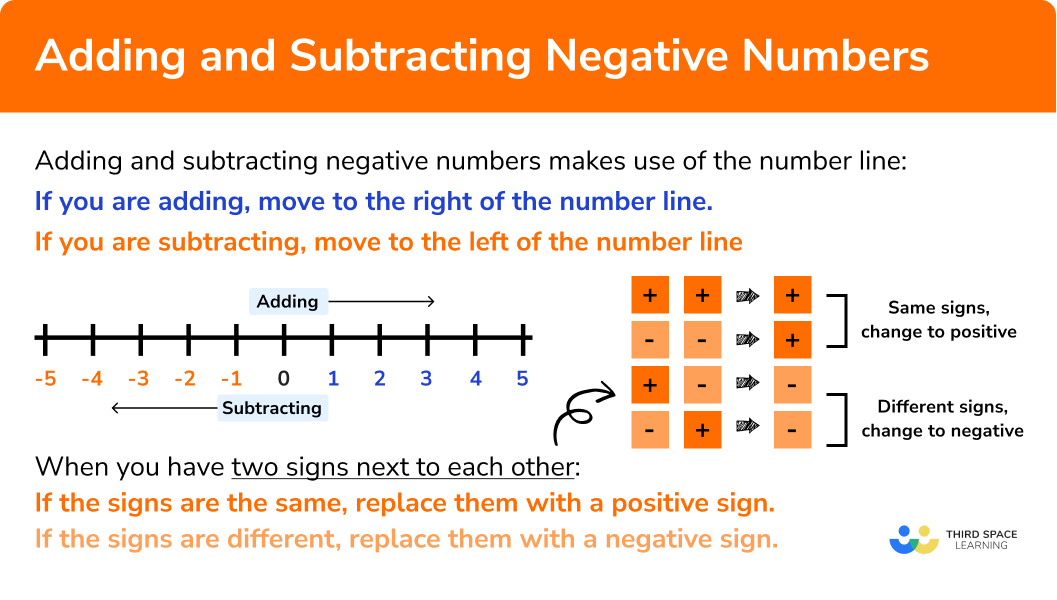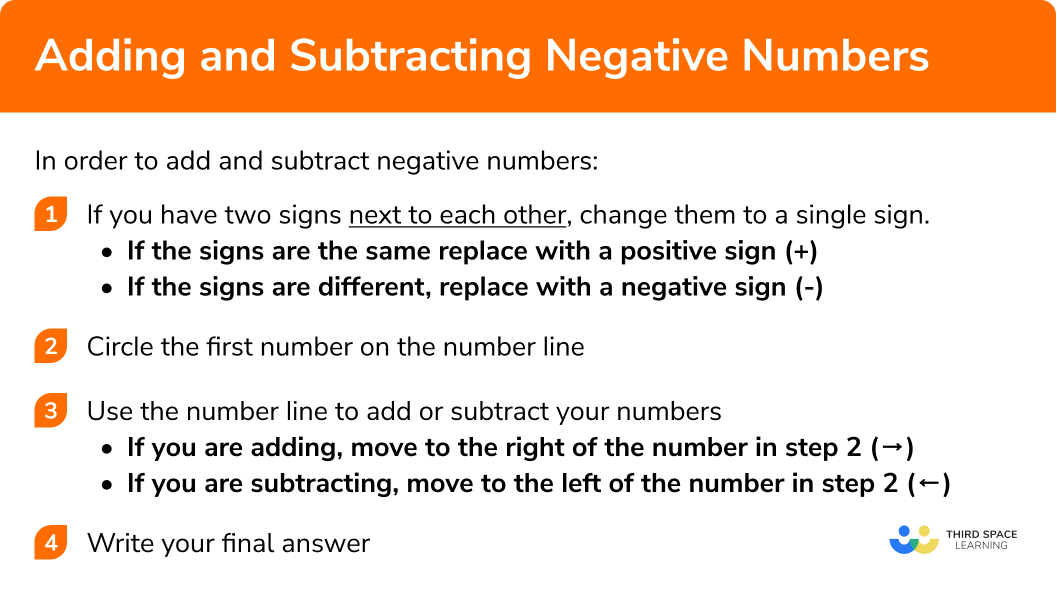GCSE Maths Number Negative Numbers

# Adding And Subtracting Negative Numbers

Here we will learn about adding and subtracting negative numbers including what negative numbers are and how to add and subtract them.

There are also negative numbers worksheets and exam questions at worksheets based on Edexcel, AQA and OCR exam questions, along with further guidance on where to go next if you’re still stuck.

## What are negative numbers?

Negative numbers are any numbers less than zero and that have a negative sign (−) in front of them.

Numbers greater than zero are referred to as positive numbers. If there is no sign in front of a number the number is positive.

On the number line below the numbers in orange are negative values and the blue numbers are positive values:

Just like you can add and subtract positive numbers, you can do the same with negative numbers.

When adding and subtracting negative numbers use a number line:

If you are adding, move to the right of the number line.

If you are subtracting, move to the left of the number line.

When you have two signs next to each other:

If the signs are the same replace with a positive sign.

If the signs are different, replace with a negative sign.

### What do you need to remember when adding and subtracting negative numbers?## How to add and subtract negative numbers

In order to add and subtract negative numbers:

1. If you have two signs next to each other, change them to a single sign.
If the signs are the same replace with a positive sign (+).
If the signs are different, replace with a negative sign (−).
2. Circle the first number on the number line.
If you are adding, move to the right of the number in step 2 (→).
If you are subtracting, move to the left of the number in step 2 (←).

### Explain how to add and subtract negative numbers in 4 steps## Adding and subtracting negative numbers examples

### Example 1: adding a positive number

$-4+7$

1. If you have two signs next to each other, change them to a single sign.
If the signs are the same replace with a positive sign (+).
If the signs are different, replace with a negative sign (−).

In this case you do not have two signs next to each other.

2Circle the first number on the number line.

The first number in the question is (−4)

If you are adding, move to the right of the number in step 2 (→).
If you are subtracting, move to the left of the number in step 2 (←).

In this case we are adding the 7 so move 7 spaces right from the (−4) on the number line:

$-4 + 7 = 3$

### Example 2: adding a negative number

$-2+(-3 )$

In this case you have a plus and a minus next to each other.

Since the signs are different, replace with a minus sign (−):

$-2 -3$

The first number in the question is (−2)

In this case we are subtracting 3 so move 3 spaces left from the −2 on the number line:

$-2 + (-3) = -5$

### Example 3: subtracting a positive number

$-5-2$

In this case you do not have two signs next to each other.

The first number in the question is (−5)

In this case we are subtracting 2 so move 2 spaces left from the (−5) on the number line:

$-5 – 2 = -7$

### Example 4: subtracting a negative number

$-8-(-10)$

In this case you have a minus and a minus next to each other.

Since the signs are the same, replace with a plus sign (+)

$-8 + 10$

The first number in the question is (−8)

In this case we are adding 10 so move 10 spaces right from the (−8) on the number line:

$-8 – (-10) = 2$

### Example 5: mixed operations

$7-8 – (-5)$

In this case you have a minus and a minus next to each other.

Since the signs are the same, replace with a plus sign (+)

$7 – 8 + 5$

The first number in the question is (7)

In this case we are subtracting positive 8 so move 8 spaces left from the 7 on the number line:

Now we are adding 5 so move 5 spaces right from the (−1) on the number line:

$7 – 8 – (-5) = 4$

### Example 6: worded question

Alina had £12 in her bank account. She purchased a coat costing her £20. By how much has she overdrawn?

Let’s start with 12 as the first number of the equation. Since she spent 20 the money is coming out of her bank account so you have to subtract it.

$12 – 20$

In this case you do not have two signs next to each other.

The first number in the question is (12)

In this case we are subtracting 20.

Because the scale on the number line below is in 2s, move 10 spaces left (20 ÷ 2 = 10)  from the 12 on the number line:

$12 – 20 = -8$

She is overdrawn by £8.

### Common misconceptions

• Greater negative does not mean a larger number

A common error is to assume that the larger a negative number is, the bigger number is.
E.g.
−3 is smaller than 2

Adding and subtracting negative numbers is part of our series of lessons to support revision on negative numbers. You may find it helpful to start with the main negative numbers lesson for a summary of what to expect, or use the step by step guides below for further detail on individual topics. Other lessons in this series include:

### Practice adding and subtracting negative numbers questions

1. Work out: -6 + 10

-44-16162. Work out: -4 + -8

-12124-4There is a + - together so change it to a -
-4 + - 8 = -4 - 83. Work out: -9 – 12

-3321-214. Work out: -15 – (-6)

-21-9921There is a - - together so change it to a +
-15-(-6) = -15+65. Work out: -7 + (-8) -5+2

-2-8-18-12There is a + - together so change it to a -
-7+(-8)-5+2 = -7 -8 -5 +26. At 5 am in the morning, the temperature in Cambridge was -9 ℃. By 10 am the temperature had risen by 11 ℃. What was the new temperature?

20^{\circ}\text{C}-20^{\circ}\text{C}-2^{\circ}\text{C}2^{\circ}\text{C}The calculation we need to do is  -9+11### Adding and subtracting negative numbers GCSE questions

The table shows the temperature in Watford at different times of the day.

 Time of the Day Temperature  (℃) 2am -3 4am 0 6am 2 8am 6

(a) Write down the lowest temperature.

(b) Find the difference between the warmest and the coldest temperature readings.

(3 marks)

−3

(1)

Identifying the warmest and coldest temperatures, 6 and -3

(1)

6−3=9

(1)

2. Sarah has the following 6 cards:She is going to choose 2 cards and subtract them.

(a) What is the largest possible number she can make?
(b) Sarah now decided to add the numbers rather than subtract them. What is the smallest possible number she can make?

(4 marks)

a) for identifying 3 or −8 or 3− (−8) seen

(1)

correctly subtracting two numbers or 11 seen

(1)

b) for identifying −7 or −8

(1)

correctly adding two numbers or −15 seen

(1)

3. Mr. and Mrs. Smith had £ 156.78 in their bank account. At the end of the month they had to pay 4 bills. They paid the telephone bill of £ 67.20 , utilities of £ 34.78 , car insurance £ 78.24 , and credit card bill of £ 144 . How much were the Smiths overdrawn?

(3 marks)

Finding the sum of the 4 bills
(£324.22)

(1)

Subtracting the cost of the four bills from the balance in the bank account
(£156.78-£324.22)

(1)

£ 167.44

(1)

## Learning checklist

You have now learned how to:

• Add and subtract integers both positive and negative
• Use negative numbers in context and calculate intervals across 0

## Still stuck?

Prepare your KS4 students for maths GCSEs success with Third Space Learning. Weekly online one to one GCSE maths revision lessons delivered by expert maths tutors.

Find out more about our GCSE maths tuition programme.